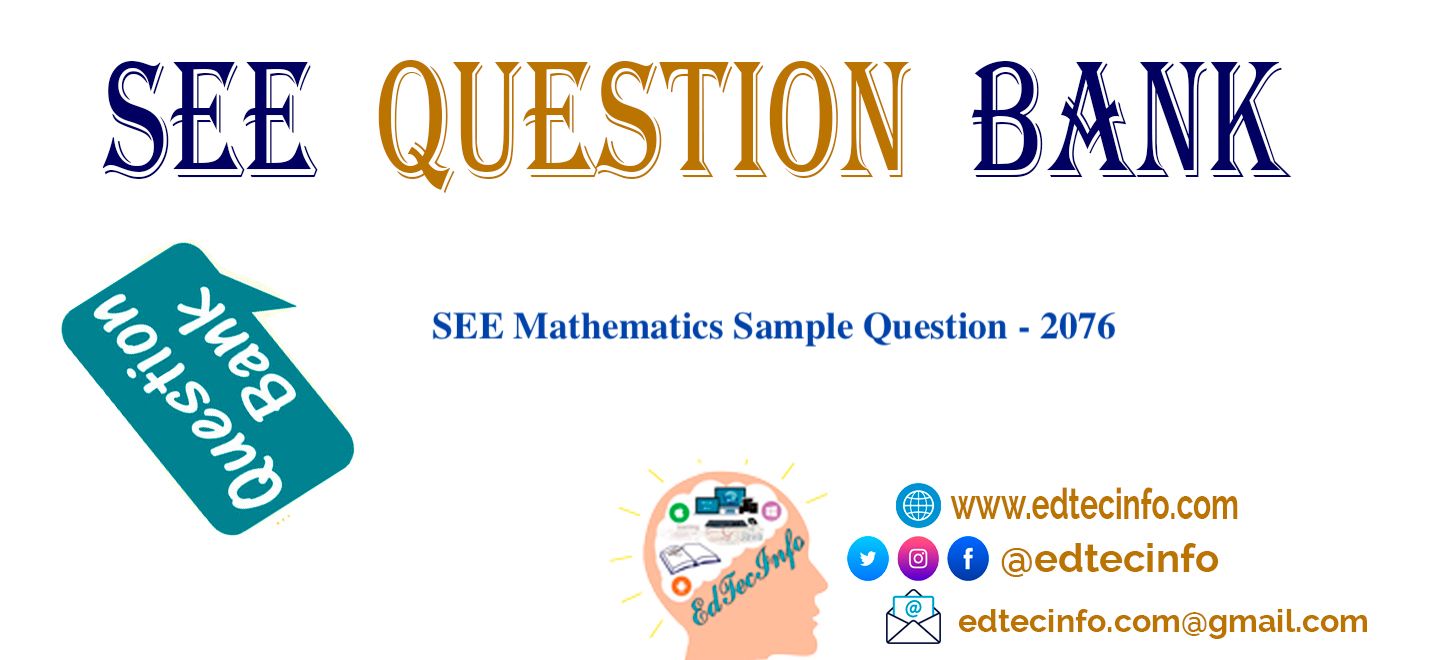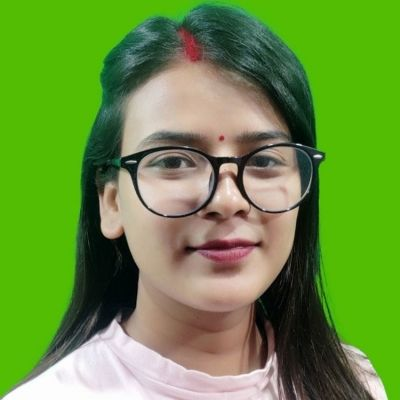### SEE Mathematics Sample Questions – 2076 Set: B

2020-Apr-13Group: A (6x1=6)

1. What is the VAT amount, if selling price is Rs 4000 and VAT% is 13%?
2. The base of an isosceles triangle is b cm and equal sides are a cm. what is the area of the triangle.
3. Simplify:
4. If the lower limit of the median class of any grouped data is L, frequency of that class is f, class interval i,
sum of the frequencies N and the cumulative frequency of pre-median class is cf, then write the formula for
finding the median.
5. Write the relation between the area of a rectangle and a triangle standing on the same base and between the
same parallels.
Scroll below for more questions: